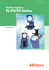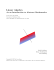# UBLUE FOR THE REGULAR TWO-STAGE LINEAR MODEL FROM

## Transcription

UBLUE FOR THE REGULAR TWO-STAGE LINEAR MODEL FROM
```Electronic Journal of Applied Statistical Analysis
EJASA (2013), Electron. J. App. Stat. Anal., Vol. 6, Issue 1, 97 – 109
e-ISSN 2070-5948, DOI 10.1285/i20705948v6n1p97
© 2013 Università del Salento –http://siba-ese.unile.it/index.php/ejasa/index
UBLUE FOR THE REGULAR TWO-STAGE LINEAR MODEL FROM
THE PERSPECTIVE OF PROJECTION OPERATORS
Surendra P. Sinha(1)*, Arnaldo Goitía(1), Jorge L. Valera(2)
(1)
(2)
Departamento de Matemática y Física, Universidad Nacional Experimental del Táchira, Táchira,
Venezuela
Received 02 July 2011; Accepted 10 December 2012
Available online 26 April 2013
Abstract:The linear models in multi stages and in particular the two-stage
models, have great potential that may be used in many scientific research.
Nevertheless more than 20 years have elapsed since the two-stage model was
defined by  and still the use of these models is not yet popular among applied
research workers, which we attribute to the complicated expressions of the
estimators of the mean vector and other parameters of the model obtained till
now in the different papers published on this subject. This article gives
alternative expressions for UBLUE of  and  in the regular two-stage linear
model, using projection operators onto ℳ() and a linear transformation F of
the observable random vector y which is linearly sufficient in order to provide
new insights and facilitate the use of these models in applied research.
Keywords:UBLUE, two-stage lineal model, Projector, transformed model.
1.
Introduction
The statistical study of linear models is an area of much interest because of its usefulness in the
analysis of data in different fields of the human knowledge. According to Graybill , a general
linear model is  =  +  where  is an observable random vector,  is an observable matrix,
is a vector of unknown parameters and  is an unobservable random vector with   =  and
= . The different variants of a model are related with the specification of the
distributional properties of , or , or with the specification of the assumptions about the
structure of the matrix , or of the matrix .
*
Email: [email protected]
97
UBLUE for the regular two-stage linear model from the perspective of projection operators
At present, the case when the matrix  = ! , is widely known and used by many researchers
from various disciplines. For this case, the BLUE of  is given by  =  ′ ! ′  =
where  =  ′ ! ′ is the perpendicular projection operator onto the column space of
ℳ  parallel to (or along) ℳ  , with  = ! which is a matrix of maximum rank such
that ′  = . In the case  = !  with  positive definite, the BLUE of  is
=  ′!!  ! ′ !!  =   and again  ′!!  ! ′ !! is the projection operator on
ℳ  parallel to ℳ  . In both cases we see that the estimator  is a projection of the
response vector  onto ℳ  .
The two-stage and p-stage models were defined by Kubáck in  and  respectively. Such
models have also been investigated by Volaufova in several of her articles, particularly , 
and . Volaufova  gives explicit but rather complicated expressions for the LBLUE
(Locally best linear unbiased estimate) of β1, β2 and establishes that the UBLUE obtained from
the transformed model exists if and only if ℳ() ⊂ ℳ(! ) , and in this case the LBLUE’s are
UBLUE’s.
Although it has been more than 20 years since the definition of two-stage linear model was given
by Kubáck , the use of this model by most researchers in different areas of human
knowledge has been almost negligible which we attribute to the complicated expressions of the
estimators of the mean vector and other parameters of the model obtained till now in the different
papers published on this subject. We think that in order to facilitate the use of the great potential
possessed by these models in the practical applications, it will be necessary to obtain equivalent
expressions of the parameters vector of the model using the results published by Bhimasankaram
and Sengupta  and Rao  so that the estimators obtained can be expressed as functions of the
projection operators.
We will use the following notation: ′ is the transpose of . ! denotes any generalized inverse
(g-inverse) of  as defined by Rao , i.e. ! is such that !  = . ! is a matrix of
maximum rank such ′! = . ℳ() is the vector space generated by the columns of the
matrix .  = (′)! ′ is the perpendicular projection operator onto ℳ() and  =
(′)! ′ where  is a positive definite matrix, is a projection operator onto ℳ(). |
is a projection operator onto ℳ() parallel to (or along) ℳ() as defined by Rao .
In this paper we use the definition of UBLUE (Uniformly best linear unbiased estimator) given
by Wulff and Birkes , which says that for the model (y, ,  ) where  is a vector of
unknown parameters of fixed effects and  a positive definite matrix, which depends on a
vector of parameters  that belongs to a known , a linear estimator ′  is called UBLUE if it
is the BLUE of its expectation for all  ∈ .
2.
Let:
The regular two-stage linear model
! = ! ! + !
! = ! +  ! + !
98
Sinha, S.P., Goitía, A., Valera, J.L. (2013). Electron. J. App. Stat. Anal., Vol. 6, Issue 1, 97 – 109.
be a model where ! and ! are random vectors with dimensions ! ×1, ! ×1 respectively;
! , ! and  are known matrices of real numbers; ! , ! are vectors of unknown parameters of
dimension ! ×1, ! ×1; and ! , ! are random error vectors with  ! = ,  ! = !! ! ,
! = ,  ! = !! ! , ! and ! uncorrelated, !! > 0, !! > 0 unknown parameters and
! , ! are known positive definite matrices of proper dimension. This model is denominated as
two-stage linear model and according to Kubácěk  if the ranks of the matrices ! , ! , ! , !
are  ! = ! < ! ,  ! = ! < ! ,  ! = ! ,  ! = ! , then the
model is
denominated as a regular two-stage linear model.
The regular two-stage linear model can be written as:
!
!
=
!

!
!
+
! !
!
(1)
=  +  with
=
σ!! !

σ!! !
=  where ! , ! are of full rank by columns, ! , ! are positive definite matrices and !! > 0,
!! > 0 unknown parameters. When  in the model (1) is pre multiplied by the  matrix where:
=
!
−

!
then, we obtain the transformed model as:
!
!
∗ =

!
!
+
! !
−
∗ = ∗  + ∗  !
! !
(2)
with
∗ = ∗ =
σ!! !
−σ!!
−σ!! ′
σ!! !!! ! + σ!! !
where ∗ = , ∗ = , ∗ = , ∗ = −! + ! , ∗ = −! + ! ,  is a matrix such
that ! = ! , ! an identity matrix, and  = ! .
Volaufova  gives explicit expressions although a little complicated, for the LBLUE (Locally
best linear unbiased estimate) of ! , ! obtained from the transformed model (2), and
establishes that the UBLUE of ! , ! obtained from the transformed model exists if and only if
ℳ() ⊂ ℳ(! ), and in this case the LBLUE’s are UBLUE’s.
99
UBLUE for the regular two-stage linear model from the perspective of projection operators
3.
UBLUE for X*β
In this paper we are interested in getting the UBLUE for  from another perspective. For doing
this, we will use the theory given by Bhimasankaram and Sengupta . Since in the model (2)
!! , !! are unknown, we will assume that the ratio  = !! !! is known, and so we obtain the
model:
!
!
∗ =
!

!
!
+ ∗
! !
!
(3)
∗ = ∗  +  ∗
with
∗ = ∗ = !! ! = !!
!
−
−′
+ !
!!! !
where  ∈ ϱ and ϱ consists of all positive real numbers. In this way, the model (3) represents a Gauss-Markov model and using the lemma (2.1) given by
Bhimasankaram and Sengupta , the BLUE of ∗  in the model ∗ , ∗ , ∗ = !! ! is
obtained as:
∗  =  − !  − ∗
− ∗ !  − ∗
!
− ∗ ∗
(4)
where
∗ =
!

!
and ! , ! are the perpendicular
respectively. Let:
=  −  ∗ =
then
i)
ii)
! − !

projection operator matrices onto ℳ(! ), ℳ(! )

= !

! − !

!
! !
−′!
!! ′
−! !  ! + ! !

−! ′!
!  = ! ! !
!! !
−! ! ! !  ! + ! ! !
!  =
The required necessary and sufficient condition for the existence of the UBLUE’s given by
ℳ() ⊂ ℳ(! ), implies that !  = ! ! =  and in this way both expressions are
simplified to:
100
Sinha, S.P., Goitía, A., Valera, J.L. (2013). Electron. J. App. Stat. Anal., Vol. 6, Issue 1, 97 – 109.
i)
ii)
! !

−! ! !

!  = ! ! !

! ! !
!  =
In fact, from (4) we get:
∗  =
! − ! ! ! ! ! ! !
! ! ! ! ! !
!

∗
!
! − ! ! ! ! ! !
which implies that
∗  =
! − !! !
!! !

!
∗
!
! − ! !
(5)
which is the BLUE and also the UBLUE for ∗  in the model (3) as a consequence of the
necessary and sufficient condition established by Volaufova .
In this article we express ∗  as a function of an oblique projector defined by Rao  which in
this case is a projection operator onto ℳ(∗ ) parallel to ℳ(! ). In this way in a simplified
form we can write the UBLUE of ∗  as:
∗  = ∗ |!  ∗
(6)
This result is proved in the part 1 of the theorem 1.
It may be noted that the estimator ∗  given in (6) exists for all possible values de  contained
in the parametric space , and therefore according to Wulff and Birkes  we can assert that it
is the UBLUE of ∗ .
Remark 1. Observe that using the lemma (2.1) of Bhimasankaram and Sengupta , the
expression for the BLUE of ! ! obtained from the model ! = ! ! + ! where ! is of full
rank by columns,  ! = σ!! ! with ! non-singular is:
! ! = ! − !! ! ! .
Furthermore, the BLUE ! ! obtained from the model ∗! =  ! + ∗! , ∗! =  ∗! =
! − ! =  ! +  ! = σ!! ! + σ!! ! ! ! , ! of complete rank by
columns and ! non singular is:
! ! = ! − ∗!  − !
= ! − !! ! ∗!
− ! ∗!  − !
!
− ! ∗! = ! − !! ! ∗! 101
UBLUE for the regular two-stage linear model from the perspective of projection operators
4.
UBLUE of β and X*β when is  =   known
Since ! and ! are of complete rank by columns, from (6) we obtain the UBLUE for  and
given by:
= ∗! ∗  = ∗! ∗ |!  ∗ = ∗! ∗ |!
(7)
= ∗! ∗  = ∗! ∗ |!  ∗ = ∗! ∗ |!   (8)
where:
∗! =
!!

=
!
!
!! ! ! !!

!! ! ! !!
Also we get:
!!
=

! − !! !

!
− ! − !! !
!

=
!!

! − !! !
!
!
! − !! !

− ! − !! !
! − !! !
!
!
which are the estimators of  and  for the model (1) with the assumption that  = σ!! σ!! is
known. Also observe that both estimators are functions of . An equivalent expression to (8)
for the estimator  is given by:
= !! ∗ |!
since ∗! = !! ∗ ∗! and ∗ ∗! ∗ |!  = ∗ |!  .
Also from  = !  we obtain the following:
a)
∗! ∗ |!   = ∗! ∗ |!  ∗ = ∗! ∗ = .
b)
∗! ∗ |!    = ∗! ∗ |!   !  = ∗! ∗ |!  ∗  =
σ!! ∗! ∗ |!  !  = σ!! ∗!  = . Using a) and b) we observe that ∗! ∗ |!   (or equivalently !! ∗ |!  ) is a projection
operator onto ℳ() parallel to ℳ( ).
Also the estimators (7) and (8) are UBLUE’s for  and  respectively for the model (1) which
are functions of the estimators in (6) when  = σ!! σ!! is known.
102
Sinha, S.P., Goitía, A., Valera, J.L. (2013). Electron. J. App. Stat. Anal., Vol. 6, Issue 1, 97 – 109.
5.
UBLUE of β and X*β for all  > ,  >
The UBLUE for  in the model (1) for all σ!! > 0, σ!! > 0 can be obtained from the UBLUE of
∗  in the model (2) using ∗ in (4) and a similar procedure which was applied to obtain the
UBLUE of  from model (3). Using the results given in the previous sections we prove the
following theorem:
Theorem 1. Consider the two-stage regular linear model given in (1). If the condition ℳ() ⊂
ℳ(! ) is satisfied then:
(i)
The projector onto ℳ(∗ ) parallel to (or along) ℳ(! ) is:
∗ |!  =
(ii)
! − !! !
!! !

.
! − !! !
The BLUE of ∗  obtained from model (3) is given by:
∗  = ∗ |!  ∗ .
(iii)
The BLUE ∗  = ∗ |!  ∗ is also the UBLUE of ∗ .
(iv)
The UBLUE’s of  and  for the model (1) when  = σ!! σ!! is known, are
respectively.
=  ∗!  ∗ |
= ∗! ∗ |!  .
(v)
The UBLUE’s of  and  for the model (1) for all !! > 0, !! > 0 are:
= ∗! ∗ |∗
= ∗! ∗ |∗
which are equal to the corresponding estimators in the case when  = σ!! σ!! is known.
(vi)
The projector onto ℳ() parallel to (or along) ℳ( ) is:
∗! ∗ |∗  .
Proof.
(i)
To show that:
∗ |!
! − !! !
=
!! !

! − !! !
103
UBLUE for the regular two-stage linear model from the perspective of projection operators
is a projection operator onto ℳ(∗ ) parallel to (or along) ℳ(! ) is a consequence of the
verification of the necessary and sufficient conditions given by Rao  in the lemma (2.4).
According to these conditions, for a disjoint partition ∗ : !  , ∗ |!  is a projector onto
ℳ(∗ ) parallel to (or along) ℳ(! ) if and only if the followings are satisfied:
a)
b)
∗ |!  ∗ = ∗
∗ |!  !  =
which can be verified using the matrix products given below:
a)
b)
! − !! !
!! !
! − ′! !

! − !! !

′! !
′! !
! −
!

= !
!

!
!
−
−′
!
!! ′
!  + !

=
!

(ii) This result is obtained by applying the expression (4) of the BLUE of ∗  (which is a
special case of the part (b) of theorem 3.2 given by Rao ) to the model (3) under the
condition ℳ() ⊂ ℳ(! ), as indicated by Volaufova  in the theorem 1.2, in the same
way as it was done in the pages 4 and 5 of this paper.
(iii) Since the BLUE ∗  = ∗ |!  ∗ of ∗  obtained in the part (ii) of this theorem exists for
all  ∈ , therefore according to Wulff and Birkes  it is also the UBLUE of ∗ .
Another way to prove this result consists in considering that according to theorem 1.2 of
Volaufova  the UBLUE of vector  = (!! , !! )′ of the model (3) exists if and only if
ℳ() ⊂ ℳ(! ), which is the condition used together with the equation (4) in order to
obtain the BLUE of ∗  of the model (3) in the part (ii) of this theorem and since
∗! ∗  = ∗!  ∗  = ∗! ∗  = , it follows that  = ∗! ∗  and ∗  = ∗ .
Therefore  and ∗  are related linearly and since  = ∗! ∗  is the UBLUE of  for
the model (3), it follows that ∗  is also the UBLUE for ∗ .
(iv) To prove this result we observe that the model (3) is a linear transformation of the model (1)
assuming that  = σ!! σ!! is known. This transformation does not affect the vector of
parameters  of the model (3), consequently  = ∗! ∗  is the estimator UBLUE of  for
both models. Therefore the estimator UBLUE of  for the model (1) can be written as
= ∗! ∗  = ∗! ∗ |  ∗ = ∗! ∗ |  . In order to obtain the UBLUE  for the
model (1) assuming  = σ!! σ!! known the matrix  is premultiplied to the estimator
= ∗! ∗  to obtain  =  = ∗! ∗  = ∗! ∗ |  .
(v) Using a similar process as the one used to find the estimator ∗  = ∗ |  ∗ given in (6)
but with ∗ of the model (2) instead of ! and considering again the condition ℳ() ⊂
ℳ(! ), we obtain the expression:
∗
104
! − !! !
=
!! !

!
∗
!
! − ! !
Sinha, S.P., Goitía, A., Valera, J.L. (2013). Electron. J. App. Stat. Anal., Vol. 6, Issue 1, 97 – 109.
which is the same as given in (5). In consequence the BLUE of ∗  for the model (2) for all
!! > 0, !! > 0 is equal to the same which was obtained with  = σ!! σ!! known in the
model (3). Since it estimator does not depend explicitly of the parameters σ!! , σ!! of the
model, ∗  given en (6) is also the BLUE of ∗  for the model (2) for all !! > 0, !! > 0
and according to Wulff and Birkes  it is also the UBLUE of ∗ . We will denote by
∗ |∗  the matrix:

∗ |∗
! − !! !
=
!! !

! − !! !
for the case of the model (2) for all !! > 0, !! > 0. In consequence the UBLUE of ∗
for this model is ∗  = ∗ |∗  ∗ .
In this way the estimators of  and  of the model (1) can be obtained as functions of  in
the same way as it was done in the proof of the part (iv) of this theorem. In this case the
estimators of  and  for the model (1) for all !! > 0, !! > 0 are given by:
= ∗! ∗  = ∗! ∗ |∗  ∗ = ∗! ∗ |∗
=  = ∗! ∗  = ∗! ∗ |∗  ∗ = ∗! ∗ |∗
(vi) In the parts a) and b) on the page 7 of this paper it was shown that:
∗! ∗ |!   =
∗! ∗ |!    =
Now from the proof of the part 5 of this theorem we know that ∗ |!  = ∗ |∗  , in
consequence:
∗! ∗ |∗   =
∗! ∗ |∗    =
and therefore ∗! ∗ |∗   is a projector onto ℳ() parallel to (or along) ℳ( ).
It may be noted that the model (2) is a nonsingular transformation of the model (1) and that the
expression for  is a linear function of  and because it is a BLUE (and also UBLUE), and so
it follows by the definition 3.1 given by Drygas  that  is a sufficient linear transformation.
Remark 2. The results obtained in this paper can also be derived using the expression (6) given in
the Theorem 3.2 of Rao . In this case the matrices  ,  are of maximum rank such that
! = !! , ! = !! .
105
UBLUE for the regular two-stage linear model from the perspective of projection operators
6.
Application
We will present an example of the application of the regular two stage model using the data
obtained from the web page of U.N.E.T. (Universidad Nacional Experimental de Táchira,
Táchira, Venezuela) . For this purpose we will use only the grade data of the entrance exam
and the preparatory courses of the high school graduates who wished to follow a career in
Mechanical, Electronic, Informatics or Civil Engineering of the U.N.E.T. in the period 2009-1
and 2010-1. From each period a random sample of sample size 40 was taken. Since the data
belongs to a group of students who followed a preparatory course and took admission exam in
different and not consecutive periods, we will assume independence among the different groups
of data. This assumption is required for the correct application of the two stage regular model.
In particular, ! = CEA2009_1 y ! = CEA2010_1 are the dependent variables in each of the
two stages of the model corresponding to the grades obtained by the aspirants in the admission
exam for the periods 2009-1 y 2010-1 respectively. The matrices ! y ! consists of two
columns of data in each case where the first column consists of the one´s corresponding to the
intercept and the second column is the explicatory variable. In this case ! = , !! ,
! = , !! where !! = CP2009_1, !! = CP2010_1 are the explicatory variables in the
stage 1 and stage 2 respectively corresponding to the preparatory course grades and  is a matrix
of the one´s of dimension 40×1. In this way ! , ! correspond with the period 2009-1 y ! , !
with the period 2010-1.
In relation to the covariance matrices we will assume that ! , ! has the pattern given by:
1

! = !
⋮
!
!
1
⋮
!
… !
… !
⋱ ⋮
… 1
for  = 1, 2 y ! = 0.3, ! = 0.3. In other words we will assume that the elements within each
sample are equally correlated. In order that the condition ℳ() ⊂ ℳ(! ) is satisfied, we will
write  = !  where  has the dimension ! ×! . For this example we will use:
=
0
0
0
1
Other possibilities for  may be:
=
1
0
0
,
1
=
1
0
0
.
0
In this way the two stage model can be written as:
! = ! ! + !
! = ! ! + ! ! + !
106
Sinha, S.P., Goitía, A., Valera, J.L. (2013). Electron. J. App. Stat. Anal., Vol. 6, Issue 1, 97 – 109.
or equivalently:
! = !!  + !" !! + !
∗
∗
! = !"  + (!" + !! ) + ! = !"
+ !!
+ !
where, ! = !! , !" ′, ! = !" , !! ′. In this case the term !" represents the influence of
the stage 1 on the stage 2. The data corresponding to each period is tabulated in Table 1.
Table 1. Sample data used for the estimation of model parameters.
Period
Obs
1
2
3
4
5
6
7
8
9
10
11
12
13
14
15
16
17
18
19
20
2009-1
y1
X11
44,69 5,50
57,33 36,75
67,86 47,17
44,57 16,67
49,25 17,58
51,79 23,67
52,32 28,33
41,03 6,42
46,25 13,33
58,26 27,50
46,80 12,17
49,19 16,17
54,68 29,17
61,92 27,08
48,04 18,08
57,76 36,92
44,38 9,58
56,58 13,50
40,62 7,50
47,67 18,58
2010-1
y2
X22
47,08 33,25
41,37 31,67
45,41 35,08
38,87 33,33
37,74 26,42
42,67 39,25
36,92 23,67
31,56 25,00
44,57 32,17
46,96 37,83
33,42 25,42
38,20 31,42
41,33 28,92
43,58 42,42
46,42 34,83
37,17 28,67
47,53 41,17
49,67 32,00
33,50 20,75
37,14 29,00
Obs
21
22
23
24
25
26
27
28
29
30
31
32
33
34
35
36
37
38
39
40
2009-1
y1
X11
49,61 16,00
52,79 12,00
51,65 15,50
61,72 15,08
55,93 22,67
52,83 36,33
40,68 9,08
50,83 19,25
47,50 10,42
46,25 13,33
49,42 22,92
51,17 20,75
42,73 2,17
42,57 5,33
49,54 16,42
44,63 10,67
52,53 14,75
46,87 15,92
53,75 14,00
58,63 32,58
2010-1
y2
X22
34,83
28,50
48,75
36,42
46,70
41,08
37,76
29,75
41,50
25,75
46,01
36,08
49,01
37,50
42,51
27,58
39,94
24,67
30,18
25,75
38,18
24,75
41,67
17,17
35,17
23,58
33,50
19,67
32,79
21,08
33,40
23,00
33,40
23,00
38,37
22,17
47,79
41,08
39,05
21,92
The results obtained by filling the two stage model are presented in Table 2 in which we observe
∗
∗
that !"
= !" y !!
= !" + !! . A measure of the influence of the stage 1 on the stage 2
represented by !" = 0.4934, may be interpreted as a measure of the influence of the factors
related to the preparatory course which may change as the correction process to improve this
course is carried out. In this sense, the influence of the preparatory course of the period 2010_1
(CP2010_1) over the grade of the admission exam for the same period (CEA2010_1), can be
expressed as the sum of two components. One component associated to factors inherent in the
preparatory course represented by 0.4934*CP2010_1 and the component related to the factors
related to the knowledge of the student 0,1257*CP2010_1. The total influence of the grade of the
preparatory course of the period 2010_1 on the admission exam is given by the sum of these two
components and is equal to 0.61907*CP2010_1.
107
UBLUE for the regular two-stage linear model from the perspective of projection operators
Table 2. Regression coefficients obtained by fitting a two-stage model.
= !
Stage 1 coefficients
!!
!"
41.4771
0.4934
Stage 2 coefficients
∗
∗
!!
!"
!!
21.9851 0.1257 21.9851 0,61907
!"
This method of interpreting the regression coefficients reflects the reality as regards the
deficiency of the necessary knowledge with which most of the students at present are admitted to
the venezuelan universities. In this way the model corresponding to the second stage can be
expressed as CEA2010_1! = 21,9851 + 0.61907 CP2010_1! ,  = 1, 2, … , 40.
7.
Conclusion
The estimation theory of multi-stage linear models and particularly two stage linear model is
known for more than twenty years, still the use of these models is very rare in applied research
which we attribute to the lengthy and complicated expressions of the parameter estimators of the
mean vector and other parameters of the model as published in different papers on this subject.
In this article, equivalent alternative expressions for the UBLUE of ,  and ∗  as functions
of projector operators onto ℳ() and ℳ(∗ ) in the untransformed and transformed version of
the regular two-stage linear model, are obtained which should facilitate and provide new
insights for the use of these models in applied research.
References
. Bhimasankaran, P., Sengupta, D. (1996). The linear zero functions approach to linear
models. Sankhy: The Indian Journal of Statistics, Series B, 58(3), 338-351.
. Drygas H. (1983). Sufficiency and Completeness in the General Gauss-Markov Model.
Sankhy: The Indian Journal of Statistics, Series A, 45(1), 88-98.
. Graybill, F.A. (1976). Theory and Application of the Linear Model. Duxbury Press, North
Scituate, MA.
. Kubácek, L. (1986). Multistage regression model. Applications of Mathematics, 31(2), 8996.
. Kubácek, L. (1988). Two-stage regression model. Mathematica Slovaca, 38(4), 383-393.
. Rao C.R. (1967). Calculus of generalized inverse of matrices, Part I: General Theory.
Sankhy, Series A, 29(3), 317-341.
. Rao C.R. (1974). Projectors, Generalized Inverses and the BLUE's. Journal of the Royal
Statistical Society. Series B, 36(3), 442-448.
. Universidad Nacional Experimental del Táchira (2012). Curso Propedéutico. URL:
. Volaufova, J. (1987). Estimation of parameters of mean and variance in two-stage linear
models. Applications of Mathematics, 32(1), 1-8.
. Volaufova, J. (1988). Note on the estimation of parameters of the mean and the variance in
n-stage. Applications of Mathematics, 33(1), 41-48.
108
Sinha, S.P., Goitía, A., Valera, J.L. (2013). Electron. J. App. Stat. Anal., Vol. 6, Issue 1, 97 – 109.
. Volaufova, J. (2004). Some estimation problems in multistage. Linear Algebra and its
Applications, 388, 389-397.
. Wulff, S., Birkes, D. (2005). Best unbiased estimation of fixed effects in mixed ANOVA
models. Journal of Statistical Planning and Inference, 133, 305-313.
This paper is an open access article distributed under the terms and conditions of the Creative Commons
Attribuzione - Non commerciale - Non opere derivate 3.0 Italia License.
109
```

#### Similar documents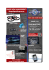### Types of Projections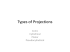### BASIC STATISTICS 1. S , R S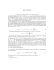### Outline: I. Spatial reference systems and projections. II. Geographic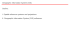### Robust Inference in Sample Selection Models Mikhail Zhelonkin , Marc G. Genton### Map Projections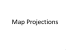### Document 6529925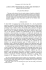### Map Projections Map Projection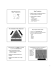### Regression Analysis of Data from a Cluster Sample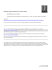### Preface to Secrets Of Shastiamsa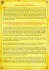### fulltext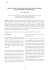### Brochure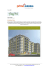### ESTIMATING SPECIES RICHNESS: SENSITIVITY TO SAMPLE COVERAGE U B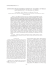### Gerardus Mercator 1512 – 1594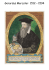### gis-driven classification of satellite imagery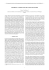### Blending World Map Projections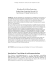### PROFILE PROJECTORS PJ-H30 Series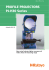### IN TERIA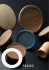### Map projections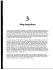### Profile Projector PJ/PV/PH Series